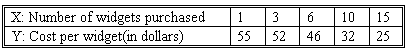### CFA Practice Question

There are 208 practice questions for this study session.

### CFA Practice Question

We are interested in finding the linear relation between the number of widgets purchased at one time and the cost per widget. The following data has been obtained:Suppose the regression line is YHAT = -2.5X + 60. We compute the average price per widget if 30 are purchased and observe YHAT = -15 dollars:

A. obviously we are mistaken; the prediction YHAT is actually +15 dollars.
B. this is obvious nonsense. The regression line must be incorrect.
C. this is obvious nonsense. This reminds us that predicting Y outside the range of X values in our data is a very poor practice.